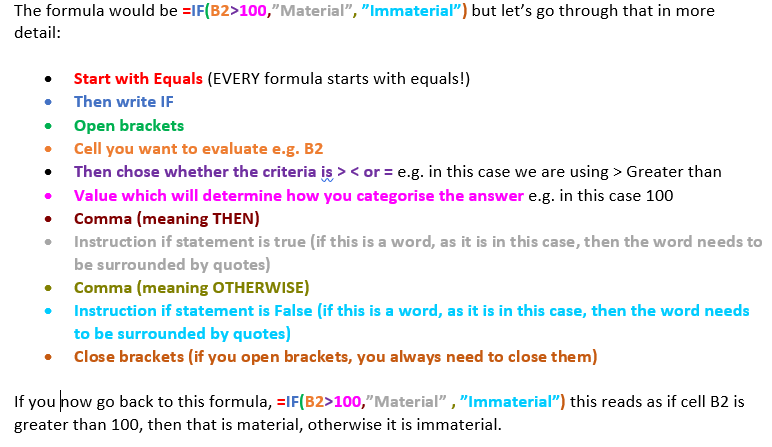# How to use an IF Formula

## How to use an IF formula in Excel

Do you need Excel IF Formula help?

An IF function is a great way of categorising your data. For example, if a cell is greater than zero it could be classified as profit, or if a cell is less than eg £100 then classify as immaterial.

In some cases, a basic IF formula will not be enough to get the results you require, an IF statement often needs to be accompanied by an OR formula or an AND formula. You might also need to combine more than one IF statements together. This is called a nested IF, in other words, an IF within an IF! You also might want to classify your values so that they can then easily be analysed in a pivot table.

But let’s start with a basic IF to start with.

Let’s use an example of classifying a value as either material or immaterial. Material will be anything greater than £100, therefore by default, if it is not material, it will be immaterial.

The cell which you are analysing is in Cell B2

The formula would be =IF(B2>100,”Material”,”Immaterial”) but let’s go through that in more detail:

• Start with Equals (EVERY formula starts with equals!)
• Then write IF
• Open brackets
• Cell you want to evaluate e.g. B2
• Then chose whether the criteria is > < or = e.g. in this case we are using > Greater than
• Value which will determine how you categorise the answer e.g. in this case 100
• Comma (meaning THEN)
• Instruction if statement is true (if this is a word, as it is in this case, then the word needs to be surrounded by quotes)
• Comma (meaning OTHERWISE)
• Instruction if statement is False (if this is a word, as it is in this case, then the word needs to be surrounded by quotes)
• Close brackets (if you open brackets, you always need to close them)

If you now go back to this formula, =IF(B2>100,”Material”,”Immaterial”) this reads as if cell B2 is greater than 100, then that is material, otherwise it is immaterial.

Here is a colourcoded version to help, and you could print it out!If you need Excel IF Formula help, or help with Nested IFs, or how to work with AND/OR forumlas or for Pivot table help then click here to contact us.

Unlike most Excel spreadsheet training courses - we will not be provide generic Excel spreadsheet training instead each course will be tailored to needs of the group - with the agenda set by the people attending the course.

This training is suitable for employees from all departments and levels of experience.

Training for PCs or Macs.

In Central London, The City, Canary Wharf, West End, NW London and the surrounding areas.

Training available in:

Office 365
Microsoft Excel 2016
Microsoft Excel 2013
Microsoft Excel 2011
Microsoft Excel 2010
Microsoft Excel 2007

### Meet Your Instructor, Gina CohenMicrosoft Excel & PowerPoint training and Spreadsheet Consulting specialist with eleven years experience in the Finance department at Morgan Stanley.

07887 552 045

Scroll to Top